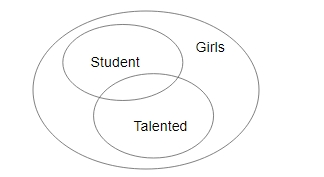### SSC JE Electrical Engineering 2015 Question 7

Instructions

In following questions one/two statement(s) are given are followed by two conclusion/assumption, I and II. You have to consider the statements to be true even if they seem to be at variance from commonly known facts. You have to decide which of the given conclusion/assumptions, if any, follows from the given statements.

Question 7

# Statements : All students are girls.Some students are not talented.Conclusions : I. No students is talentedII. Some girls are talented

SolutionNeither I nor II follows.

$$\therefore$$ the correct answer is option D.